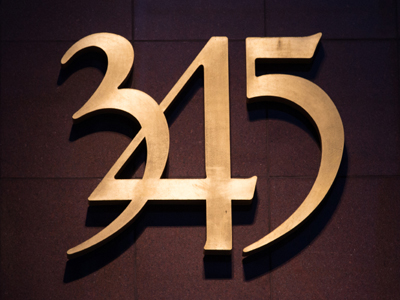'Trebled', 'quadrupled' and 'quintupled' mean multiply by 3, 4 and 5.

# Multiplication and Division 2 (Very Easy)

This is the second of our very easy Eleven Plus maths quizzes on multiplication and division. In it you’ll learn some techniques and strategies that will make your calculations even easier to do. Try all the quizzes in our very easy section before moving up a level.

One thing which will help you no end when dividing or multiplying numbers, is knowing all your times tables off by heart. That way you’ll avoid having to rely on calculators or pencils and paper. I mean, you wouldn’t want to get either of those out if you were in a shop trying to work out how much something will cost you to buy, would you?

The questions here are quite simple. Nevertheless, you’d be wise to read each of them carefully before selecting your answer. Get used to doing that now and it’ll be second nature when you take your exams.

1.
What is the correct answer to the given calculation?
16 trebled
4
8
32
48
'Trebled' means multiplied by 3
2.
What is the correct answer to the given calculation?
174 ÷ 100
0.174
1.74
17.4
0.0174
To divide by 100, simply move the decimal point two places to the left. Remember that, in whole numbers, the decimal point comes after (i.e. 174 is the same a s 174.00000)
3.
What is the correct answer to the given calculation?
36 x 20
576
648
720
900
To multiply by 20, first multiply by 2 then by 10:
36 x 2 = 72, then 72 x 10 = 720
4.
What is the correct answer to the given calculation?
750 ÷ 5
150
75
300
250
To swiftly divide by 5, first divide by 10 then double your answer:
750 ÷ 10 = 75, then 75 x 2 = 150
5.
What is the correct answer to the given calculation?
999 × 11
1,089
10,890
10,989
110,889
There are two quick ways to work this one out. First, to multiply by 999, times by 1,000 then subtract the original number:
11 x 1,000 = 11,000. Then 11,000 - 11 = 10,989.
To quickly multiply by 11, first times by 10, then add the original number:
999 x 10 = 9,990. Then 9,990 + 999 = 10,989
6.
What is the correct answer to the given calculation?
28
34
51
68
7.
What is the correct answer to the given calculation?
1.8 x 400
720
7,200
72
7.2
To multiply by 400, first multiply by four, then by 100:
1.8 x 4 = 7.2, then 7.2 x 100 = 720
8.
What is the correct answer to the given calculation?
1,000,000 (1 million) ÷ 2,000
50
100
500
1,000
To quickly divide by 2,000, first divide by 1,000, then divide your answer by 2:
1,000,000 ÷ 1,000 = 1,000, then 1,000 ÷ 2 = 500
9.
What is the correct answer to the given calculation?
28 × 99
252
2,772
532
3,052
A quick way to multiply by 99 is to first multiply by 100, then subtract the original number:
28 x 100 = 2,800, then 2,800 - 28 = 2,772
10.
What is the correct answer to the given calculation?
250 quintupled
500
750
1,000
1,250
'Quintupled' means multiplied by five. A quick way to work this problem out is to first multiply by 10, and then divide by 2:
250 x 10 = 2,500, then 2,500 ÷ 2 = 1,250
Author:  Frank Evans